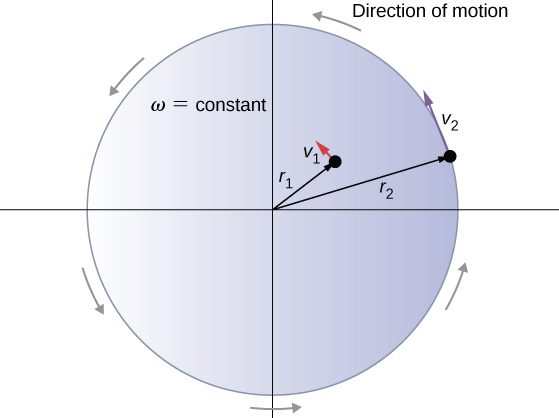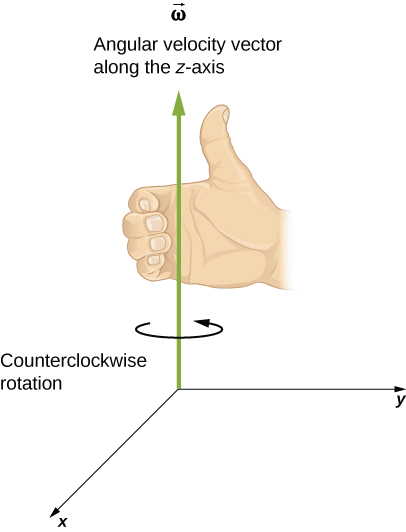# 10.1 Rotational variables  (Page 2/11)

 Page 2 / 11

where $\theta$ is the angle of rotation ( [link] ). The units of angular velocity are radians per second (rad/s). Angular velocity can also be referred to as the rotation rate in radians per second. In many situations, we are given the rotation rate in revolutions/s or cycles/s. To find the angular velocity, we must multiply revolutions/s by $2\pi$ , since there are $2\pi$ radians in one complete revolution. Since the direction of a positive angle in a circle is counterclockwise, we take counterclockwise rotations as being positive and clockwise rotations as negative.

We can see how angular velocity is related to the tangential speed of the particle by differentiating [link] with respect to time. We rewrite [link] as

$s=r\theta .$

Taking the derivative with respect to time and noting that the radius r is a constant, we have

$\frac{ds}{dt}=\frac{d}{dt}\left(r\theta \right)=\theta \frac{dr}{dt}+r\frac{d\theta }{dt}=r\frac{d\theta }{dt}$

where $\theta \frac{dr}{dt}=0$ . Here $\frac{ds}{dt}$ is just the tangential speed ${v}_{\text{t}}$ of the particle in [link] . Thus, by using [link] , we arrive at

${v}_{\text{t}}=r\omega .$

That is, the tangential speed of the particle is its angular velocity times the radius of the circle. From [link] , we see that the tangential speed of the particle increases with its distance from the axis of rotation for a constant angular velocity. This effect is shown in [link] . Two particles are placed at different radii on a rotating disk with a constant angular velocity. As the disk rotates, the tangential speed increases linearly with the radius from the axis of rotation. In [link] , we see that ${v}_{1}={r}_{1}{\omega }_{1}$ and ${v}_{2}={r}_{2}{\omega }_{2}$ . But the disk has a constant angular velocity, so ${\omega }_{1}={\omega }_{2}$ . This means $\frac{{v}_{1}}{{r}_{1}}=\frac{{v}_{2}}{{r}_{2}}$ or ${v}_{2}=\left(\frac{{r}_{2}}{{r}_{1}}\right){v}_{1}$ . Thus, since ${r}_{2}>{r}_{1}$ , ${v}_{2}>{v}_{1}$ .Two particles on a rotating disk have different tangential speeds, depending on their distance to the axis of rotation.

Up until now, we have discussed the magnitude of the angular velocity $\omega =d\theta \text{/}dt,$ which is a scalar quantity—the change in angular position with respect to time. The vector $\stackrel{\to }{\omega }$ is the vector associated with the angular velocity and points along the axis of rotation. This is useful because when a rigid body is rotating, we want to know both the axis of rotation and the direction that the body is rotating about the axis, clockwise or counterclockwise. The angular velocity $\stackrel{\to }{\omega }$ gives us this information. The angular velocity $\stackrel{\to }{\omega }$ has a direction determined by what is called the right-hand rule. The right-hand rule is such that if the fingers of your right hand wrap counterclockwise from the x -axis (the direction in which $\theta$ increases) toward the y- axis, your thumb points in the direction of the positive z -axis ( [link] ). An angular velocity $\stackrel{\to }{\omega }$ that points along the positive z -axis therefore corresponds to a counterclockwise rotation, whereas an angular velocity $\stackrel{\to }{\omega }$ that points along the negative z -axis corresponds to a clockwise rotation.For counterclockwise rotation in the coordinate system shown, the angular velocity points in the positive z- direction by the right-hand-rule.

We can verify the right-hand-rule using the vector expression for the arc length $\stackrel{\to }{s}=\stackrel{\to }{\theta }\phantom{\rule{0.2em}{0ex}}×\phantom{\rule{0.2em}{0ex}}\stackrel{\to }{r}$ , [link] . If we differentiate this equation with respect to time, we find

$\frac{d\stackrel{\to }{s}}{dt}=\frac{d}{dt}\left(\stackrel{\to }{\theta }\phantom{\rule{0.2em}{0ex}}×\phantom{\rule{0.2em}{0ex}}\stackrel{\to }{r}\right)=\left(\frac{d\stackrel{\to }{\theta }}{dt}\phantom{\rule{0.2em}{0ex}}×\phantom{\rule{0.2em}{0ex}}\stackrel{\to }{r}\right)+\left(\stackrel{\to }{\theta }\phantom{\rule{0.2em}{0ex}}×\phantom{\rule{0.2em}{0ex}}\frac{d\stackrel{\to }{r}}{dt}\right)=\frac{d\stackrel{\to }{\theta }}{dt}\phantom{\rule{0.2em}{0ex}}×\phantom{\rule{0.2em}{0ex}}\stackrel{\to }{r}.$

a length of copper wire was measured to be 50m with an uncertainty of 1cm, the thickness of the wire was measured to be 1mm with an uncertainty of 0.01mm, using a micrometer screw gauge, calculate the of copper wire used
Mustapha
If centripetal force is directed towards the center,why do you feel that you're thrown away from the center as a car goes around a curve? Explain
Which kind of wave does wind form
calculate the distance you will travel if you mantain an average speed of 10N m/s for 40 second
hw to calculate the momentum of the 2000.0 elephant change hunter at a speed of 7.50 m/s
how many cm makes 1 inches
2.5
omwoyo
2.54cm=1inche
omwoyo
how do we convert from m/s to km/hr
When paddling a canoe upstream, it is wisest to travel as near to the shore as possible. When canoeing downstream, it may be best to stay near the middle. Explain why?
Explain why polarization does not occur in sound
one ship sailing east with a speed of 7.5m/s passes a certain point at 8am and a second ship sailing north at the same speed passed the same point at 9.30am at what distance are they closet together and what is the distance between them then
density of a subtance is given as 360g/cm,put it in it s.i unit form
if m2 is twice of m1. find the ration of kinetic energy in COM system to lab system of elastic collision
What is a volt equal to?
list and explain the 3 ways of charging a conductor
conduction convention rubbing
Asdesaw
formula of magnetic field
why polarization does not occur in sound
Integral of a vector
define surface integral of a vector?
Rahat

#### Get Jobilize Job Search Mobile App in your pocket Now!ByBy OpenStaxBy OpenStaxBy JavaChamp TeamBy OpenStaxBy RhodesBy Edgar DelgadoBy Brooke DelaneyBy JavaChamp TeamBy David Corey